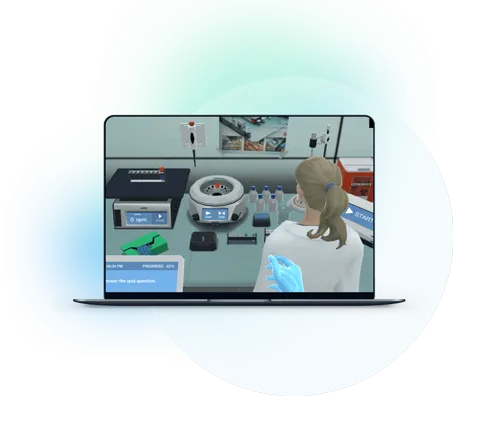Blog

# 5 Ways to Get Students Energized About Scalars and Vectors

Teaching with Labster
October 11, 2022

All measurable quantities are termed physical quantities.

We deal with numerous such quantities daily. Some of these quantities just need a numerical value for their description i.e. length, mass, temperature, time, etc. These quantities are called scalars. However, in some cases, we need to mention a direction along with a numerical value i.e. when mentioning force, displacement, or velocity. All these quantities are vectors.

Vectors have played a great role in solving complex physical problems. They have helped engineers design bridges buildings, machines, and complex systems. In short, vectors are the basis of engineering mechanics.

If you’re teaching a physics or basic mechanics course, you will probably start with scalars and vectors. However, some students may find this topic hard.

Read on to learn why the concept can be tricky, and then use five ways through which you can make scalars and vectors an approachable topic.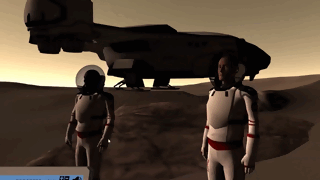GIF from Labster's Vector and Scalar simulation.

## Why the concepts of Scalars and Vectors are tricky?

Physics is usually easy for students, however, some topics like that scalar and vectors can be tricky for students to understand for the following three reasons.

### 1. Differentiating between the two is hard

Physical quantities are measurable quantities that can be either vectors or scalars. Students often fail to understand which one is a scalar and which one is a vector. Another thing that makes it hard for them is when you say velocity is a vector quantity, however its magnitude (speed) is scalar.  A thorough explanation is required for clearing their misconceptions.

### 2. Sketching vectors graphically can be tricky

Sketching off vectors graphically in the cartesian and polar coordinates is tough for students. This becomes more difficult when you are dealing with a 3D plane. Moreover, vectors do have components along the cartesian planes. So, first drawing a vector graphically and then splitting it into its components is a lot for students to digest.

### 3. Vector Calculations are Different

Since vector comes with directions, correctly representing them is another problem students have to face. Moreover, the addition, subtraction, and multiplication of vectors are not based on ordinary mathematical laws. They are added and subtracted graphically through head-to-tail laws. Moreover, vector multiplication is completely different from scalar multiplication. And there is no such thing as vector division.

## 5 Ways to Make Scalars and Vectors Interesting

Keeping in view some of the problems faced by students, here are five ways through which you can introduce them to scalars and vectors in an interesting way.

### 1. Differentiate between two terms

You can talk about scalars and vectors, one by one. However, to make it easier for students, construct a table of comparison between two quantities. The more differences you quote, the easier it becomes to distinguish between the two quantities.

Scalars & Vectors

A scalar quantity just possesses magnitude i.e. a numerical value. A vector quantity possesses magnitude as well as direction.

A scalar quantity is one-dimensional.Vector quantity is muti-dimensional.

Scalar quantity changes when its magnitude changes.Vector quantity changes when either its direction or magnitude changes.

Scalar doesn’t have any components.Vectors can have multiple components depending on the Cartesian Plane.

Multiplication of two scalars gives a scalar.Multiplication of two vectors can result in a scalar quantity (dot product) as well as a vector quantity (cross product).

Examples are speed, distance, length, mass, and volume.Examples are force, velocity, weight, and displacement.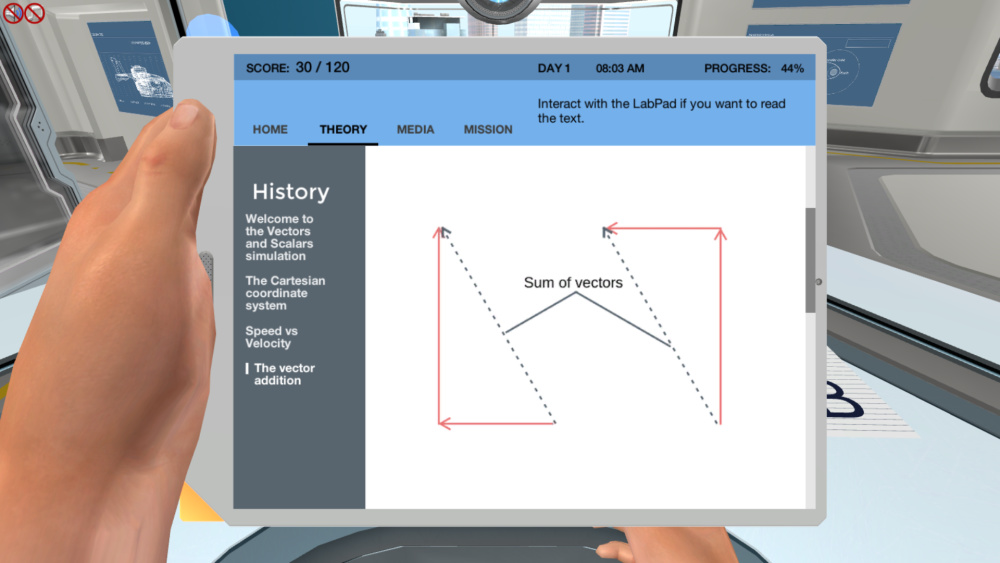From Labster's simulation: Vector and Scalar.

### 2. Tell Them How to Represent Vectors and Split it

Another trouble students face is representation. Since the direction is involved, we represent vectors graphically in a coordinate system.

Cartesian and polar coordinate systems are usually used. However, for representing vectors, we normally prefer the cartesian plane. Further, a cartesian plane can be two-dimensional or three-dimensional. For simplicity purposes, you should start with a two-dimensional coordinate system. For constructing a two-dimensional Cartesian coordinate system, construct two perpendicular lines; label the horizontal one as the x-axis and the vertical one as the y-axis.

You can be provided a vector in two ways, either the magnitude of a vector with orientation would be given, or the coordinates of a vector would be present.

For instance, if you are asked to represent a 20 N force directed at an angle of 45 degrees with the horizontal. Then, you can follow these steps:

1. Draw two perpendicular lines, and label them as x and y-axis.

2. Set up a suitable scale to represent that vector on paper. For instance, you can say 1 cm represents 4 N on the paper. That means you will need a 5 cm line to represent 20 N.

3. Using a protractor and a ruler, draw a 5 cm line, starting from the origin, at an angle of 45’ with respect to the positive axis.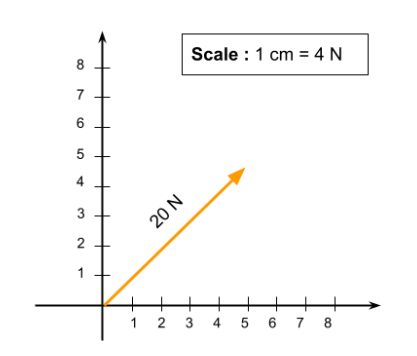In another case, if you are given coordinates of one or two vectors. Just map those points on the Cartesian plane, and then join the points to the origin to form a vector. For instance, you are given two points, (1, 5), and (3, 4), and asked to represent vectors.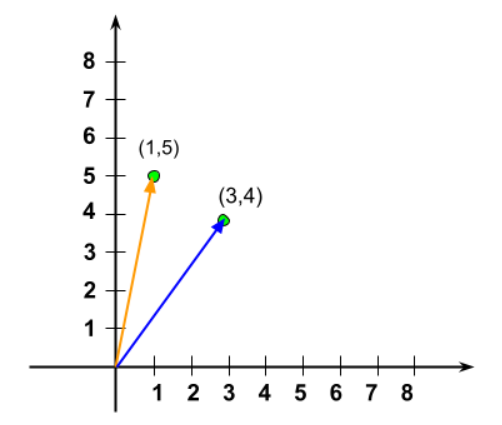Once you tell students how to draw vectors, you can explain to them the process of splitting the vector into its components. For instance, we have a force vector F in the cartesian plane. We can split this force into its perpendicular components. For that, just draw perpendicular lines from the head of the vector to each axis. Now, form a vector along the x-axis, it is Fcos . And another vector along the y-axis is Fsin .  These two vectors are the components of force in both directions.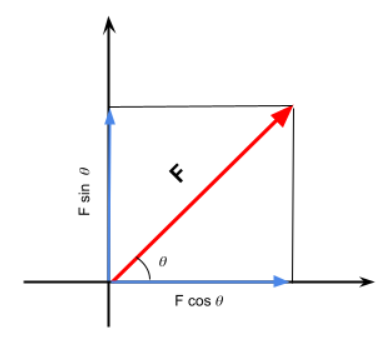Vector resolution is quite helpful for us. If a force is acting diagonally at any angle, then we can find out its magnitude in the horizontal and vertical directions.  This makes the problem simpler, as the addition of perpendicular components is quite easy,

### 3. Explain How Vector Calculations are Different from Scalars

Students are often confused about vector calculations. Unlike scalar quantities, vectors do have a direction, so they cannot be added or subtracted like scalars. One way to add the vectors is graphical. You are given two or more vectors on a Cartesian plane, and you have to add them.

The vector addition is governed by the head-to-tail rule, which is very simple if you pay attention to the figure illustrated below. For instance, we have two vectors, A and B, in the cartesian plane as shown. Now, to add vector A to vector B, we place the head of vector B on the tail of the first vector A. If you have more vectors, you will keep placing their tails on the head of preceeding vectors. Once you are finished, join the tail of the first vector with the head of the last vector.  This gives you the vector sum of all vectors.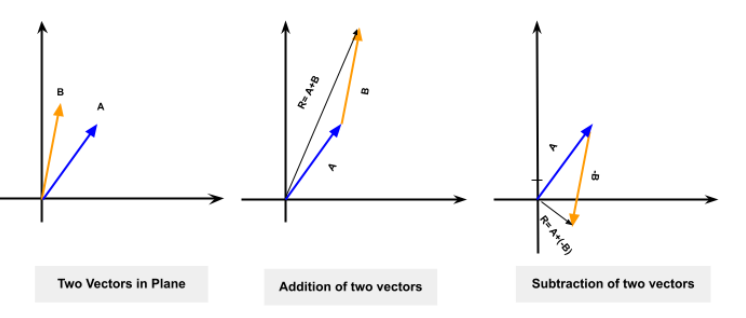Vector subtraction is similar to vector addition. For instance, to subtract vector B from vector A, take the negative of vector B (the direction is reversed) and add it to vector A, as shown in the figure.

### 4. Demonstrate with Some Practical Examples

The interesting part about teaching Physics is that you can relate it to the real world. It gives you insights into the processes around you. However, even with good points you put forward to explain scalars and vectors, students won’t fully understand until you put forward some practical examples.

Luckily, there are a lot of examples to quote. For instance, you went to a shop and asked him to give you 4 kilograms of rice; the shopkeeper understood and gave you the exact quantity. Here, the mass of the rice is a scalar quantity, as it just requires you to mention a numerical value.

Similarly, if you say the total time for this test is 10 minutes, do you need to add anything else to define time? No, because you have just mentioned the quantity explicitly. Such physical quantities which just require a magnitude (numerical value) to define, are called scalars. Length, mass, time, temperature, area, and volume, all are scalars.

However, there are some cases, when you need directions as well. For instance, nowadays you frequently use Google Maps when you put on a destination address and turn on the navigation. First, the app tells you a distance in miles or kilometers to the destination and then gives specific directions to reach the destination. Without those directions, you will be lost!

Moreover, if you ask students to push or pull a table, you are specifying direction. To push the table, they will apply some force in the direction of the table, and when they pull it they will apply force towards themselves. Since force requires a magnitude and a direction for its definition, hence it’s a vector quantity. Every physical quantity, which needs a direction along with its magnitude, for its complete description, is a vector.

### 5. Use Virtual Lab Simulation

There are a lot of experiments that can be performed regarding vectors in the labs. However, you can use virtual lab simulations if your institute lacks resources. They are a great alternative and addition to physical experimentation.

At Labster, we are committed to providing you with an interactive simulation, which contains a gamified and story-telling environment set up in a 3D world. Students learn and experiment as they progress in the simulation.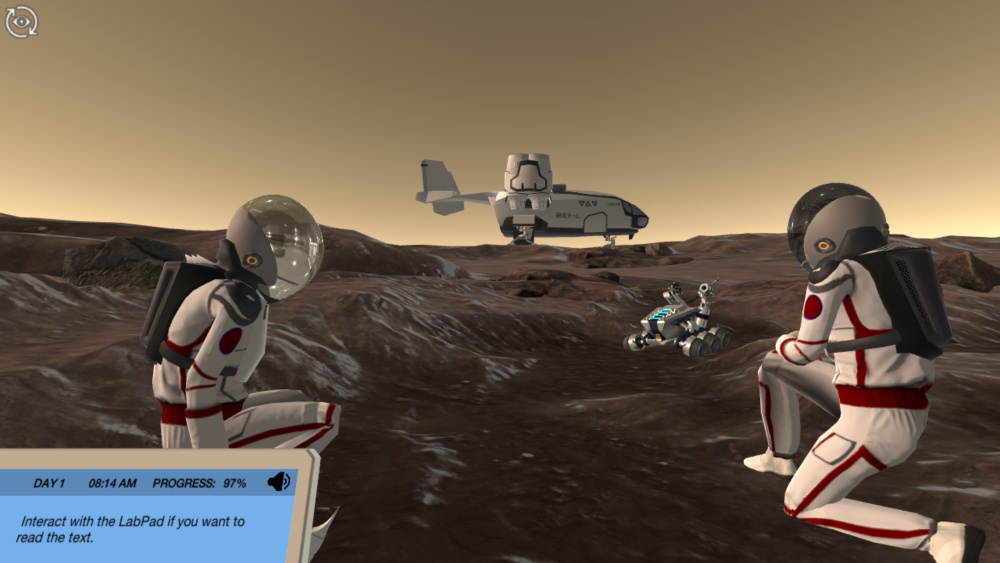You can check out our Vector and Scalar simulation, in which students guide two astronauts on a mission to Mars. Along the way, they solve basic engineering problems with the help of vectors.